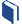# Simply Surveying

###Resources for this lesson:

> Glossary> Calculator Resources> Teacher Resources: Instructional NotesWhen presented with a data set or situation, there are some general steps you can follow to solve the problem.

1. Create and Analyze – Look at the data. Find various ways to model the mathematics, such as with a graph, table or equation. Make sense of the problem you are working with by reasoning through concepts you already know that may help you solve the problem.
2. Test and Confirm – Analyze the models and representations to develop strategies you think work best to solve the problem. You may need to try a few strategies and models until you get the one that is best. Persevere in solving the problem. Continually ask yourself if the steps you are following and what you are calculating makes sense in the context of the problem. Do not be afraid to change your strategy if necessary.
3. Apply the Model – Employ your chosen models, representations and strategies. Input the necessary data, quantitative values, etc., and perform the necessary calculations with precision. Analyze the final results. Do they make sense in the context you have?